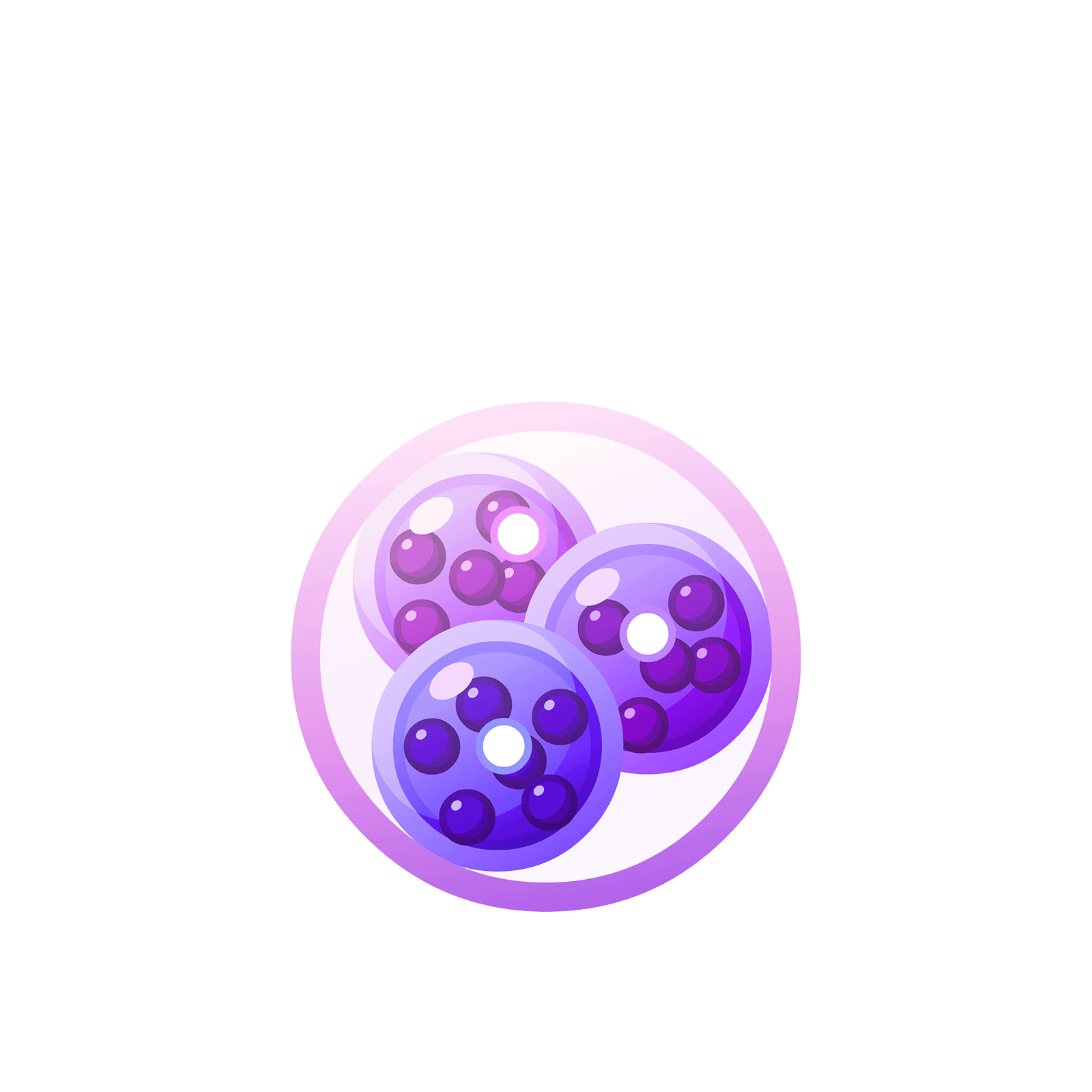06 039 290# Station  12Remainders### Question 1

Last lesson we saw that $276 \div 12$ equals $23$.Suppose we tried to compute $277\div 12$ instead. What would we see?

Let's try it!### Question 2

Compute $2789 \div 11$.### Question 3

Compute $4366 \div 14$.### Question 4

Compute $5481 \div 131$.As you play with division in dots-and-boxes you might decide that it is actually good to always work from left to right in case there are remainders: we’d like all the “extra” dots we see appear in the lower places, the ones and tens places, rather than the higher thousands places, for instance. (But even if you don’t choose to do this, you won’t go wrong! Unexplosions will always be possible to help you out.)

### Let's Go Wild!